# Midterm 2 Solution

CS 17

1. Short Answer Problems. Each of the following problems is worth 35 points.

1. For a function, define declaration, definition, call, actual parameter, and formal parameter.

• Declaration --- Also known as the prototype. The function name, return type, and number and types of parameters are made known to the compiler.
• Definition --- Where the details of the function are given; that is, the function's code is here.
• Call --- Where the function is used. Control is transferred from the calling function to the called function.
• Actual parameter --- A parameter associated with the function call. It may be any valid expression of the appropriate type.
• Formal parameter --- A parameter associated with the definition of the function. It is a local variable of the function, initialized from the value of the corresponding actual parameter.

2. For the following questions, assume the following declarations:
```int i = 1;
int j = 2;
int k = 3;
double x = 1.0;
double y = 2.0;
double z = 3.0;
```
1. Parenthesize this expression and write its value:
```i + j == k;

((i + j) == k);   ==> 1
```
2. Parenthesize this expression and write its value:
```k - i < y > x;

(((k - i) < y ) > x);   ==> 0
```
3. Parenthesize this expression and write its value:
```z - x + 1 != j + k - 2 * i;

(((z - x) + 1) != ((j + k) - (2 * i)));   ==> 0
```
4. What are the definitions of the values true and false in C++?

Any non-zero value is interpreted as ``true.'' Zero is interpreted as ``false.''

3. What is printed by the following program?
```#include <iostream.h>

int a, b, c;

void f1(void);
void f2(void);

int main()
{
int a;

a = 5;
b = 3;
c = 7;

cout << a << " " << b << " " << c << endl;
f1();
cout << a << " " << b << " " << c << endl;

return 0;
}

int d = 4;

void f1(void)
{
int c = 10;

a = 3;
b++;

cout << a << " " << b << " " << c << " " << d << endl;
f2();
cout << a << " " << b << " " << c << " " << d << endl;
}

void f2(void)
{
int d = 45;

a += 4;
b = 7;
c = d + a;
cout << a << " " << b << " " << c << " " << d << endl;
}
```

Each output value was worth 1.5 points. Here's the output:

```5 3 7
3 4 10 4
7 7 52 45
7 7 10 4
5 7 52
```

2. Programming Problems. Each of the following problems is worth 35 points. You are more likely to receive partial credit if you write your code so that I can understand what you've done.

1. Write a function for computing the number of ways in which r objects from be selected from a set of n objects (choose(n, r)). Here is the mathematical definition: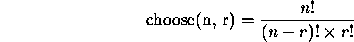The parameters n and r are integers. The function should return an integer value. Your function should make use of a factorial function, which you are also to write. Here is the definition of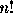: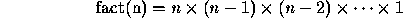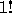and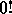are defined to have the value 1. Again, n is an integer. The function should return an integer value.

```// Prototypes.
int fact(int);
int choose(int, int);

int fact(int n)
{
int prod = 1;

while (n > 1)
{
prod *= n;
--n;
}

return prod;
}

int choose(int n, int r)
{
return fact(n) / (fact(n - r) * fact(r));
}
```

2. Write a function printFloat() with four formal parameters: number, width, fill, and precision, which prints a floating-point value, number, in scientific notation, right-adjusted in a field of width width with a fill character of fill, and a precision of precision.

```void printFloat(double number, int width, char fill, int precision)
{
cout << setw(width) << setfill(fill) << setprecision(precision)
<< setiosflags(ios::scientific) << setiosflags(ios::right)
<< value;
}
```

Thomas P. Kelliher
Thu Apr 25 22:53:59 EDT 1996
Tom Kelliher